# sigma

Singular value plot of dynamic system

## Syntax

``sigma(sys)``
``sigma(sys1,sys2,...,sysN)``
``sigma(sys1,LineSpec1,...,sysN,LineSpecN)``
``sigma(___,w)``
``sigma(___,type)``
``````[sv,wout] = sigma(sys)``````
``[sv,wout] = sigma(sys,w)``

## Description

example

````sigma(sys)` plots the singular values of the frequency response of a dynamic system model `sys`. `sigma` automatically determines frequencies to plot based on system dynamics.If `sys` is a single-input, single-output (SISO) model, then the singular value plot is similar to its Bode magnitude response.If `sys` is a multi-input, multi-output (MIMO) model with `Nu` inputs and `Ny` outputs, then the singular value plot shows `min(Nu,Ny)` lines on the plot corresponding to each singular value of the frequency response matrix. For MIMO systems, the singular value plot extends the Bode magnitude response and is useful in robustness analysis.If `sys` is a model with complex coefficients, then in:Log frequency scale, the plot shows two branches, one for positive frequencies and one for negative frequencies. The plot also shows arrows to indicate the direction of increasing frequency values for each branch. See Singular Value Plot of Model with Complex Coefficients.Linear frequency scale, the plot shows a single branch with a symmetric frequency range centered at a frequency value of zero.```

example

````sigma(sys1,sys2,...,sysN)` plots the singular values of response of multiple dynamic systems on the same plot. All systems must have the same number of inputs and outputs.```

example

````sigma(sys1,LineSpec1,...,sysN,LineSpecN)` specifies a color, line style, and marker for each system in the plot.```

example

````sigma(___,w)` plots singular values of the system response for frequencies specified by `w`.If `w` is a cell array of the form `{wmin,wmax}`, then `sigma` plots the response at frequencies ranging between `wmin` and `wmax`.If `w` is a vector of frequencies, then `sigma` plots the response at each specified frequency. The vector `w` can contain both negative and positive frequencies.You can use `w` with any of the input-argument combinations in previous syntaxes.```

example

````sigma(___,type)` plots the modified singular value responses based on the `type` argument. Specify `type` as:`1` to plot the singular values of the frequency response H-1, where H is the frequency response of `sys`.`2` to plot the singular values of the frequency response I+H.`3` to plot the singular values of the frequency response I+H-1.You can only use the `type` argument for square systems, that is, systems that have the same number of inputs and outputs.```

example

``````[sv,wout] = sigma(sys)``` returns the singular values of the response at each frequency in the vector `wout`. The output `sv` is a matrix, and the value `sv(:,k)` gives the singular values in descending order at the frequency `wout(k)`. The function automatically determines frequencies in `wout` based on system dynamics. This syntax does not draw a plot.```

example

````[sv,wout] = sigma(sys,w)` returns the singular values `sv` at the frequencies specified by `w`.If `w` is a cell array of the form `{wmin,wmax}`, then `wout` contains frequencies ranging between `wmin` and `wmax`.If `w` is a vector of frequencies, then `wout` = `w`.```

## Examples

collapse all

Create a singular value plot of the following continuous-time SISO dynamic system.

`$\mathit{H}\left(\mathit{s}\right)=\frac{{\mathit{s}}^{2}+0.1\mathit{s}+7.5}{{\mathit{s}}^{4}+0.12{\mathit{s}}^{3}+9{\mathit{s}}^{2}}$`

```H = tf([1 0.1 7.5],[1 0.12 9 0 0]); sigma(H)````sigma` automatically selects the plot range based on the system dynamics.

Create a singular value plot over a specified frequency range. Use this approach when you want to focus on the dynamics in a particular range of frequencies.

```H = tf([-0.1,-2.4,-181,-1950],[1,3.3,990,2600]); sigma(H,{1,100}) grid on```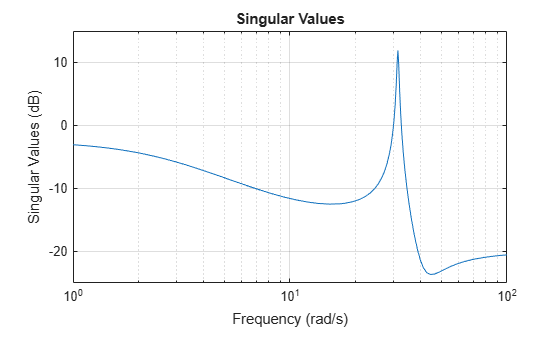The cell array `{1,100}` specifies the minimum and maximum frequency values in the plot. When you provide frequency bounds in this way, the function selects intermediate points for frequency response data.

Alternatively, specify a vector of frequency points to use for evaluating and plotting the frequency response.

```w = [1 5 10 15 20 23 31 40 44 50 85 100]; sigma(H,w,'.-') grid on````sigma` plots the frequency response at the specified frequencies only.

Compare the frequency response of a continuous-time system to an equivalent discretized system on the same singular value plot.

Create continuous-time and discrete-time dynamic systems.

```H = tf([1 0.1 7.5],[1 0.12 9 0 0]); Hd = c2d(H,0.5,'zoh');```

Create a plot that displays both systems.

```sigma(H,Hd) legend("Continuous","Discrete")```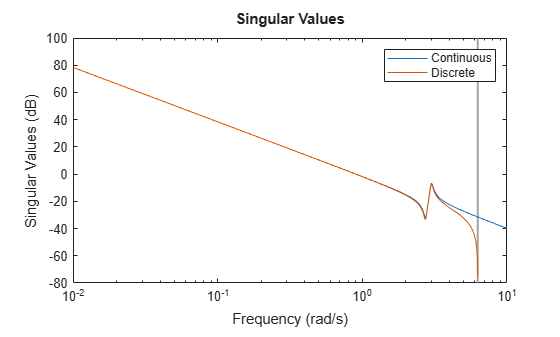The `sigma` plot of a discrete-time system includes a vertical line marking the Nyquist frequency of the system.

Specify the line style, color, or marker for each system in a `sigma` plot using the `LineSpec` input argument.

```H = tf([1 0.1 7.5],[1 0.12 9 0 0]); Hd = c2d(H,0.5,'zoh'); sigma(H,'r',Hd,'b--')```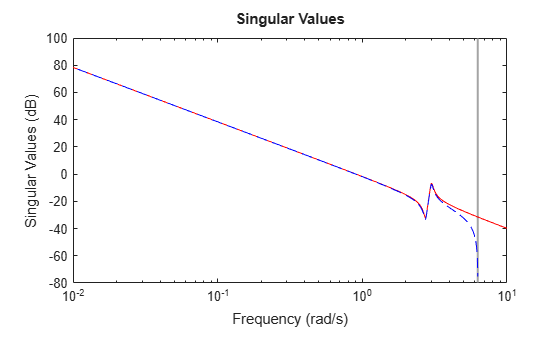The first `LineSpec`, `'r'`, specifies a solid red line for the response of `H`. The second `LineSpec`, `'b--'`, specifies a dashed blue line for the response of `Hd`.

Compute the singular values of the frequency response of a SISO system.

If you do not specify frequencies, `sigma` chooses frequencies based on the system dynamics and returns them in the second output argument.

```H = tf([1 0.1 7.5],[1 0.12 9 0 0]); [sv,wout] = sigma(H);```

Because `H` is a SISO model, the first dimension of `sv` is 1. The second dimension is the number of frequencies in `wout`.

`size(sv)`
```ans = 1×2 1 40 ```
`length(wout)`
```ans = 40 ```

Thus, each entry along the second dimension of `sv` gives the singular value of the response at the corresponding frequency in `wout`.

For this example, create a 2-output, 3-input system.

```rng(0,'twister'); % For reproducibility H = rss(4,2,3);```

For this system, `sigma` plots the singular values of the frequency response matrix in the same plot.

`sigma(H)`Compute the singular values at 20 frequencies between 1 and 10 radians.

```w = logspace(0,1,20); sv = sigma(H,w);```

`sv` is a matrix, in which the rows correspond to the singular values of the frequency response matrix and the columns are the frequency values. Examine the dimensions.

`size(sv)`
```ans = 1×2 2 20 ```

Thus, for example, `sv(:,10)` are the singular values of the response computed at the 10th frequency in `w`.

Consider the following two-input, two-output dynamic system.

`$H\left(s\right)=\left[\begin{array}{cc}0& \frac{3s}{{s}^{2}+s+10}\\ \frac{s+1}{s+5}& \frac{2}{s+6}\end{array}\right].$`

Compute the singular value responses of H(s) and I + H(s).

```H = [0, tf([3 0],[1 1 10]) ; tf([1 1],[1 5]), tf(2,[1 6])]; [svH,wH] = sigma(H); [svIH,wIH] = sigma(H,[],2);```

In the last command, the input 2 selects the second response type, I + H(s). The vectors `svH` and `svIH` contain the singular value response data, at the frequencies in `wH` and `wIH`.

Plot the singular value responses of both systems.

```subplot(211) sigma(H) subplot(212) sigma(H,[],2)```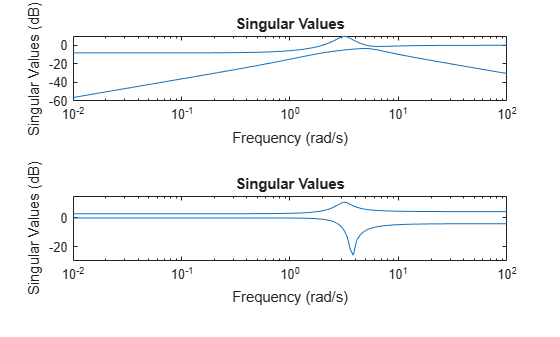Create a singular value plot of a model with complex coefficients and a model with real coefficients on the same plot.

```rng(0) A = [-3.50,-1.25-0.25i;2,0]; B = [1;0]; C = [-0.75-0.5i,0.625-0.125i]; D = 0.5; Gc = ss(A,B,C,D); Gr = rss(4); sigma(Gc,Gr) legend('Complex-coefficient model','Real-coefficient model')```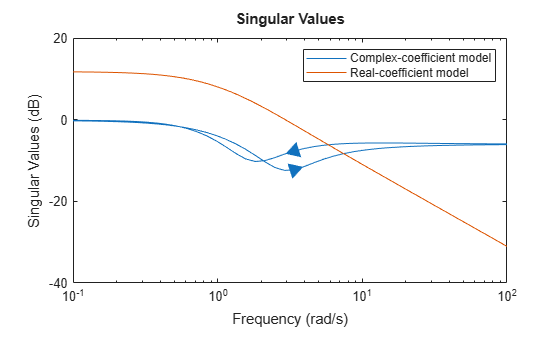In log frequency scale, the plot shows two branches for models with complex coefficients, one for positive frequencies, with a right-pointing arrow, and one for negative frequencies, with a left-pointing arrow. In both branches, the arrows indicate the direction of increasing frequencies. The plots for models with real coefficients always contain a single branch with no arrows.

You can change the frequency scale of the plot by right-clicking the plot and selecting Properties. In the Property Editor dialog, on the Units tab, set the frequency scale to `linear scale`. Alternatively, you can use the `sigmaplot` function with a `sigmaoptions` object to create a customized plot.

```opt = sigmaoptions; opt.FreqScale = 'Linear';```

Create the plot with customized options.

```sigmaplot(Gc,Gr,opt) legend('Complex-coefficient model','Real-coefficient model')```In linear frequency scale, the plot shows a single branch with a symmetric frequency range centered at a frequency value of zero. The plot also shows the negative-frequency response of a model with real coefficients when you plot the response along with a model with complex coefficients.

## Input Arguments

collapse all

Dynamic system, specified as a SISO or MIMO dynamic system model or an array of dynamic system models. Dynamic systems that you can use include:

• Continuous-time or discrete-time numeric LTI models, such as `tf`, `zpk`, or `ss` models.

• Generalized or uncertain LTI models such as `genss` or `uss` (Robust Control Toolbox) models. (Using uncertain models requires Robust Control Toolbox™ software.)

• For tunable control design blocks, the function evaluates the model at its current value for both plotting and returning frequency response data.

• For uncertain control design blocks, the function plots the nominal value and random samples of the model. When you use output arguments, the function returns frequency response data for the nominal model only.

• Frequency-response data models such as `frd` models. For such models, the function plots the response at frequencies defined in the model.

• Identified LTI models, such as `idtf` (System Identification Toolbox), `idss` (System Identification Toolbox), or `idproc` (System Identification Toolbox) models. Using identified models requires System Identification Toolbox™ software.

If `sys` is an array of models, the function plots the frequency responses of all models in the array on the same axes.

Line style, marker, and color, specified as a string or vector of one, two, or three characters. The characters can appear in any order. You do not need to specify all three characteristics (line style, marker, and color). For example, if you omit the line style and specify the marker, then the plot shows only the marker and no line. For more information about configuring this argument, see the `LineSpec` input argument of the `plot` function.

Example: `'r--'` specifies a red dashed line

Example: `'*b'` specifies blue asterisk markers

Example: `'y'` specifies a yellow line

Frequencies at which to compute and plot frequency response, specified as the cell array `{wmin,wmax}` or as a vector of frequency values.

• If `w` is a cell array of the form `{wmin,wmax}`, then the function computes the response at frequencies ranging between `wmin` and `wmax`.

• If `w` is a vector of frequencies, then the function computes the response at each specified frequency. For example, use `logspace` to generate a row vector with logarithmically spaced frequency values. The vector `w` can contain both positive and negative frequencies.

For models with complex coefficients, if you specify a frequency range of [wmin,wmax] for your plot, then in:

• Log frequency scale, the plot frequency limits are set to [wmin,wmax] and the plot shows two branches, one for positive frequencies [wmin,wmax] and one for negative frequencies [–wmax,–wmin].

• Linear frequency scale, the plot frequency limits are set to [–wmax,wmax] and the plot shows a single branch with a symmetric frequency range centered at a frequency value of zero.

Specify frequencies in units of rad/`TimeUnit`, where `TimeUnit` is the `TimeUnit` property of the model.

Option to plot modified singular values, specified as one of the following options:

• `1` to plot the singular values of the frequency response H-1, where H is the frequency response of `sys`.

• `2` to plot the singular values of the frequency response I+H.

• `3` to plot the singular values of the frequency response I+H-1.

You can only use the `type` argument for square systems, that is, systems that have the same number of inputs and outputs.

## Output Arguments

collapse all

Singular values of the frequency response in absolute units, returned as a matrix. `sv` contains the singular values computed at the frequencies `w` if you supplied them, or `wout` if you did not. For a system `sys` with `Nu` inputs and `Ny` outputs, `sv` has `min(Nu,Ny)` rows, and as many columns as there are values in `w` or `wout`.

Frequencies at which the function returns the system response, returned as a column vector. The function chooses the frequency values based on the model dynamics, unless you specify frequencies using the input argument `w`.

`wout` also contains negative frequency values for models with complex coefficients.

Frequency values are in radians per `TimeUnit`, where `TimeUnit` is the value of the `TimeUnit` property of `sys`.

## Algorithms

`sigma` uses the MATLAB® function `svd` to compute the singular values of the complex frequency response.

• For an `frd` model, `sigma` computes the singular values of `sys.ResponseData` at the frequencies, `sys.Frequency`.

• For continuous-time `tf`, `ss`, or `zpk` models with transfer function H(s), `sigma` computes the singular values of H(jω) as a function of the frequency ω.

• For discrete-time `tf`, `ss`, or `zpk` models with transfer function H(z) and sample time Ts, `sigma` computes the singular values of

`$H\left({e}^{j\omega {T}_{s}}\right)$`

for frequencies ω between 0 and the Nyquist frequency ωN = π/Ts.

## Version History

Introduced before R2006a